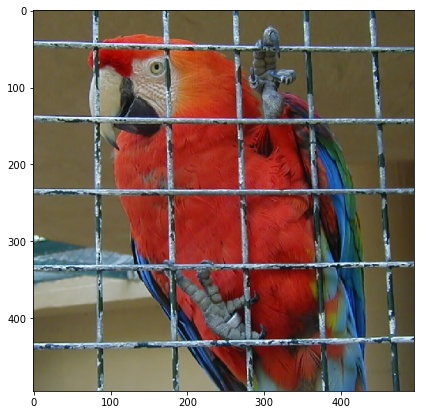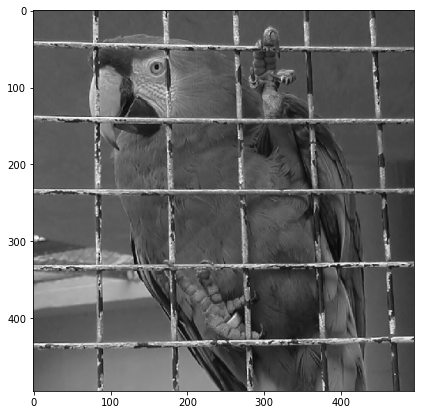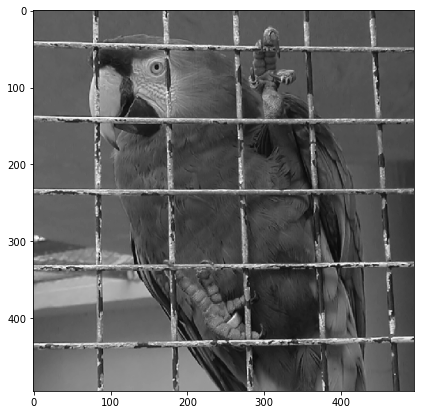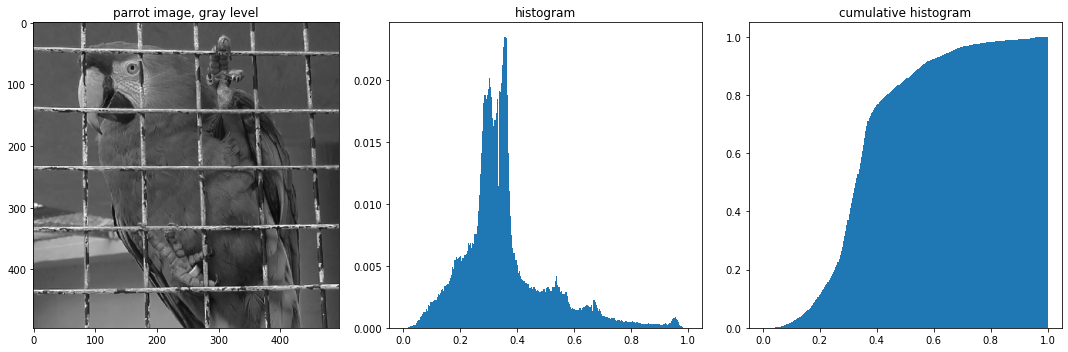# Introduction to image processing - Radiometry

## Introduction¶

For the following exercices, you need Python 3 with some basic librairies (see below). All images necessary for the session are available here.

If you use your own Python 3 install, you should download the images, put them in a convenient directory and update the path in the next cell.

In :
path = '../im/'

In :
import numpy as np
import matplotlib.pyplot as plt
%matplotlib inline


## Load and display a color image¶

A color image is made of three channels : red, green and blue. A color image in $\mathbb{R}^{N\times M}$ is stored as a $N\times M\times 3$ matrix.

Be careful with the functions plt.imread() and plt.imshow() of matplotlib.

• plt.imread() reads png images as numpy arrays of floating points between 0 and 1, but it reads jpg or bmp images as numpy arrays of 8 bit integers.

• In this practical session, we assume images encoded as floating point values between 0 and 1, so if you load a jpg or bmp file you must convert the image to float type and normalize its values.

• If 'im' is an image encoded as a double numpy array, plt.imshow(im) will display all values above 1 in white and all values below 0 in black. If the image 'im' is encoded on 8 bits though, plt.imshow(im) will display 0 in black and 255 in white.</span>

In :
imrgb = plt.imread(path+'parrot.png')


Display the image size:

In :
[nrow,ncol,nch] = imrgb.shape
print(nrow,ncol,nch)

495 495 3


You can use plt.imshow() to display the 3D numpy array imrgb as an image:

In :
plt.figure(figsize=(7, 7))
plt.imshow(imrgb)
plt.show()It might be useful to convert the color image to gray level. This can be done by averaging the three channels, or by computing another well chosen linear combination of the coordinates R, G and B. First we try with simple averaging $$I_{gs}=(R+G+B)/3$$

In :
imgray = np.mean(imrgb,2)
plt.figure(figsize=(7, 7))
plt.imshow(imgray,cmap='gray',vmin=0,vmax=1)
plt.show()1. Now use a custom weighted averaging of the three channels, that reflects better human perception: $$I_{gs}=0.21 R + 0.72 G + 0.07 B$$

In :
# to do: question 1
imgray2 = 0.21*imrgb[:,:,0] + 0.72*imrgb[:,:,1] + 0.07*imrgb[:,:,2]
# autre solution : imgray2 = np.average(imrgb,axis=2,weights=[0.21,0.71,0.07])
plt.figure(figsize=(7, 7))
plt.imshow(imgray2,cmap='gray',vmin=0,vmax=1)
plt.show()## Histograms and contrast enhancement¶

### Computing histograms¶

In the following, we compute and display the gray level histogram and the cumulative histogram of an image.

The cumulative histogram of a discrete image $u$ is an increasing function defined on $\mathbb{R}$ by $$H_u(\lambda)=\frac{1}{|\Omega|}\#{\{\textbf{x};\;u(\textbf{x})\leq \lambda\}}.$$ The histogram of $u$ is the derivative of $H_u$ in the sense of distributions.

a. We compute the histogram of the image imgray:

In :
imhisto, bins = np.histogram(imgray, range=(0,1), bins = 256)
imhisto       = imhisto/np.sum(imhisto)


b. We now compute the corresponding cumulative histogram thanks to the function np.cumsum()which cumulates the values of a vector from left to right:

In :
imhistocum = np.cumsum(imhisto)


c. We display the image, histogram and cumulative histogram:

In :
values = (bins[1:]+bins[:-1])/2
fig, axes = plt.subplots(nrows=1, ncols=3, figsize=(15, 5))
axes.imshow(imgray,cmap='gray',vmin=0,vmax=1)
axes.set_title('parrot image, gray level')
axes.bar(values,imhisto,width=1/256)
axes.set_title('histogram')
axes.bar(values,imhistocum,width=1/256)
axes.set_title('cumulative histogram')
fig.tight_layout()
plt.show()### Histogram equalization¶

If $u$ is a discrete image and $h_u$ its gray level distribution, histogram equalization consists in applying a contrast change $g$ (increasing function) to $u$ such that $h_{g(u)}$ is as close as possible to a constant distribution. We can compute directly $$H_u(u)*255.$$

To this aim, we can apply directly the vector imhistocum (which can be seen as a function from $\{0,\dots,255\}$ into $[0,1]$) to the numpy array imgray. Since imgray has values between $0$ and $1$, it is necessary to multiply it by $255$ and cast it as a 8-bit array:

In :
imgray_8bits = np.uint8(255*imgray) # changement de l'encodage des valeurs de niveaux de gris de imgray
imeq = imhistocum[imgray_8bits]


The resulting image imeq has the same shape as imgray. To better understand what operation does imhistocum[imgray_8bits], here is an example with small size matrices :

In :
a = np.array([[3,4,5],[3,1,0]]) # matrix of integers, analog to imgray_8bits
print("a=",a)
b = np.array([7,8,9,10,11,12]) # vector, analog to imhistocum
print("b=",b)
c = b[a]
print("c=",c) # c has same shape as a
# each value in a is replaced by the corresponding value : 3 becomes 10, because b=10,
# 4 becomes 11 because b=11, and so on.

a= [[3 4 5]
[3 1 0]]
b= [ 7  8  9 10 11 12]
c= [[10 11 12]
[10  8  7]]


We can now display the resulting equalized image:

In :
plt.figure(figsize=(7, 7))
plt.imshow(imeq,cmap = 'gray',vmin=0,vmax=1)
plt.show()